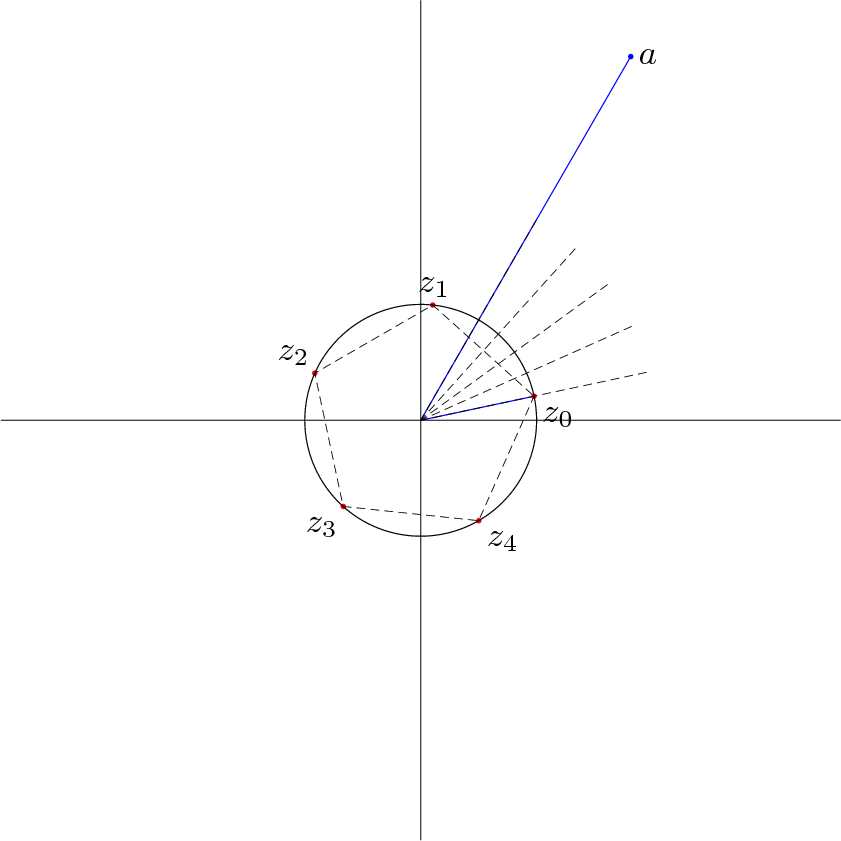Fichier 250.jps — Modifié le 2 Septembre 2006 à 22 h 04Source
``````%% gh
%% racines nème d'un complexe

60 setxunit
-5 5 setxrange
-5 5 setyrange
traceaxes

%%%%%%%%%%%%%%%%%%%%%%%%%%%%%%%%%%%%%%%%%%%%%%%
%% définitions des couleurs
%%%%%%%%%%%%%%%%%%%%%%%%%%%%%%%%%%%%%%%%%%%%%%%

/coul0 {0 0 0 0.5 setcmykcolor} def %gris
/coul1 {noir} def
/coul2 {orange} def
/coul3 {rouge} def
/coul4 {bleu} def

%%%%%%%%%%%%%%%%%%%%%%%%%%%%%%%%%%%%%%%%%%%%%%%
%% le cercle
%%%%%%%%%%%%%%%%%%%%%%%%%%%%%%%%%%%%%%%%%%%%%%%

%%%%%%%%%%%%%%%%%%%%%%%%%%%%%%%%%%%%%%%%%%%%%%%
%% les points
%%%%%%%%%%%%%%%%%%%%%%%%%%%%%%%%%%%%%%%%%%%%%%%

/n {5} def
/theta {60} def
/theta2 {theta n div} def
/rho {5} def
/rho2 {rho 1 n div exp} def

/A {theta cos rho mul theta sin rho mul} def
/B0 {theta2 cos rho2 mul theta2 sin rho2 mul} def

/rot {360 n div cos 360 n div sin} def
/B1 {B0 rot mulc} def
/B2 {B1 rot mulc} def
/B3 {B2 rot mulc} def
/B4 {B3 rot mulc} def

coul4
A point
[O A] ligne
[O B0] ligne

coul3
[B0 B1 B2 B3 B4] points

coul1
0 0 rho2 cercle

.5 setlinewidth
pointilles
[B0 B1 B2 B3 B4 B0] polygone

/rot2 {theta2 cos theta2 sin} def
/C0 {B0 2 0 mulc} def
/C1 {C0 rot2 mulc} def
/C2 {C1 rot2 mulc} def
/C3 {C2 rot2 mulc} def
/C4 {C3 rot2 mulc} def

[O C0] ligne
[O C1] ligne
[O C2] ligne
[O C3] ligne
[O C4] ligne

<latex>
\$a\$
</latex>
A [2.5 dup] crtexlabel

<latex>
\$z_{0}\$
</latex>
B0 [2.5 dup] drtexlabel
<latex>
\$z_{1}\$
</latex>
B1 [2.5 dup] uctexlabel
<latex>
\$z_{2}\$
</latex>
B2 [2.5 dup] ultexlabel
<latex>
\$z_{3}\$
</latex>
B3 [2.5 dup] dltexlabel
<latex>
\$z_{4}\$
</latex>
B4 [2.5 dup] drtexlabel``````